# Determine the force in each member of the Pratt roof truss shown. State whether each member is in tension or compression.

Question-AnswerCategory: Engineering MechanicsDetermine the force in each member of the Pratt roof truss shown. State whether each member is in tension or compression.Determine using the method of joints the force in each member of the Howe roof truss shown. State whether each member is in tension or compression.

Step: 1

Draw the free body diagram of the Howe roof truss.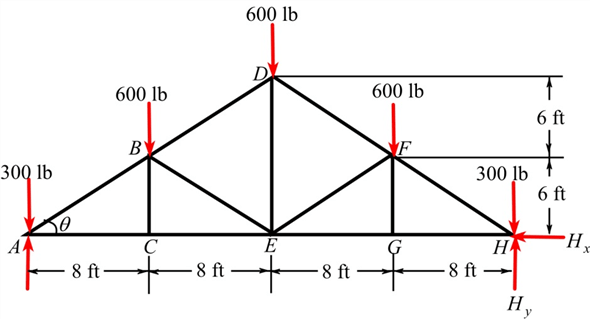Step: 2

Since the truss is symmetrical about the member, the forces in the following members are equal in magnitude.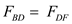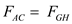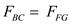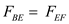Step: 3

Calculate the angleby using the following relation:Considerto be the reaction force at point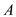due to the roller support, and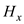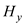to be the reactions force in the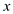anddirections respectively at point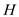due to the pin support.
Write the force equilibrium equation in thedirection.Consider the moment about point.Step: 4

Write the force equilibrium equation in the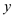direction.Substitute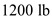for.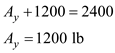Step: 5

Draw the free body diagram at joint A.Step: 6

Consider the force equilibrium equation equilibrium in thedirection.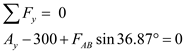Substitutefor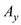.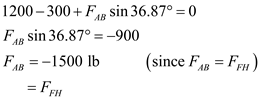Therefore, the force acting in each member AB and FH are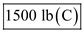.

Step: 7

Write the force equilibrium equation in thedirection.Substitute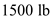for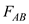.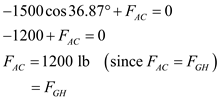Therefore, the force acting in each member AC andis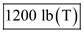.

Step: 8

Draw the free body diagram of the joint C.Step: 9

Write the force equilibrium equation in thedirection.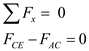Substitutefor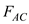.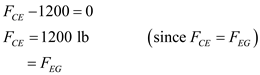Therefore, the force acting in each member CE andis.

Step: 10

Write the force equilibrium equation in thedirection.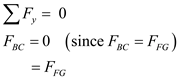Therefore, force acting in each member BC andis.

Step: 11

Draw the free body diagram of the joint B.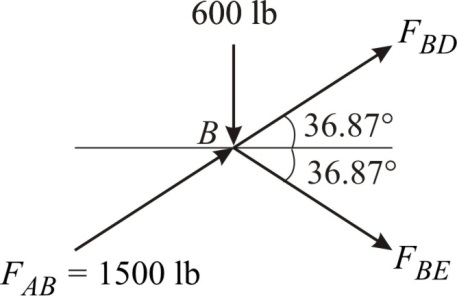Step: 12

Write the force equilibrium equation in thedirection.Substitutefor.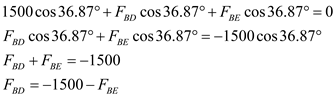…… (1)

Step: 13

Write the force equilibrium equation in thedirection.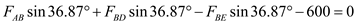Substitutefor.Substitute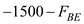for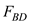.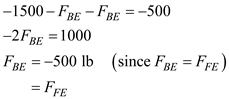Therefore, force acting in each member BE andis.
Substitute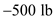forin equation (1).Therefore, the force acting in each member BD andis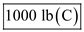.

Step: 14

Draw the free body diagram of the joint E.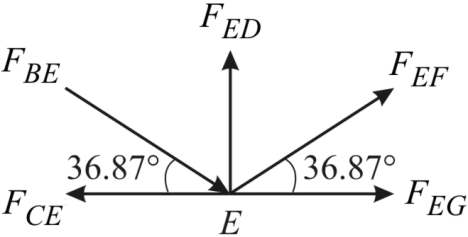Write the force equilibrium equation in thedirection.Substitutefor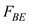andfor.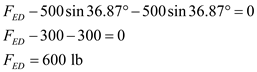Therefore, the force acting in the member ED is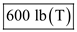.